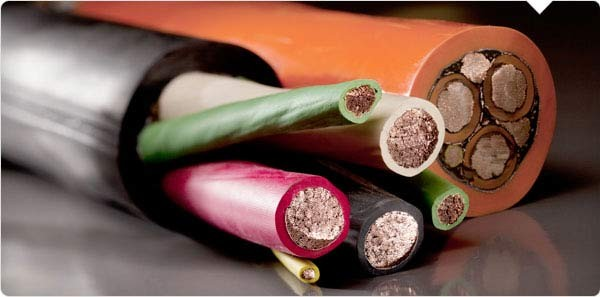# Electricity Basics## When we talk about electricity, we typically talk about three things: watts, volts and amps. These are universal units. Each one measures something different.

Electricity basics for middle school is a two-day lesson which  introduces students to circuits, watts, volts, amps, and loads.

• Watts are a basic measure of power.
• Volts are the standard unit of electromotive force. You can think of volts literally as a pushing force. The higher the voltage, the harder the electricity is being pushed through the circuit.
• Amps are the equivalent of volume. When you think about how much amperage you have available, you’re basically asking, How big is your “container” full of electricity?
• Circuit: Electricity flows in a loop called a circuit which begins and ends at the battery pack.
• Load: Any device that consumes the energy flowing through a circuit and converts that energy into work is called a load. A light bulb is an example of a load; it consumes the electricity from a circuit and converts it into work (in the form of heat and light).